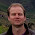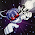## Freitag, 28. Februar 2014

### Tangent Space Normal Mapping without storing Tangents

After experimenting with the tangent space normal maps, it turned out that its possible to compute the tangent space in the vertex shader quite fast, which saves memory and bandwidth for rendering. Since the light computation is in camera space, tangent and co-tangent can be approximated as follows in the vertex shader:

VS

normal = normalize((gl_ModelViewMatrix* vec4(gl_Normal.xyz,0.0)).xyz);
tex_tan = cross(normal.xyz,vec3(0,-1,0));
tex_cotan = cross(normal.xyz,tex_tan);
tex_uv = gl_MultiTexCoord0.xy;

For the fragment shader, we need to first get the direction of the texturing by using the derivatives before we can compute the pertubed normal vector as follows:

FS

vec3 bump=vec3(-1.0,-1.0,-1.0)+2.0*texture2D(texBump,tex_uv).xyz;
vec2 tdx=normalize(dFdx(tex_uv));  // tex coord derivative in x
vec2 tdy=normalize(dFdy(tex_uv));  // tex coord derivative in y
vec3 n =bump.z*normal              // Z-direction of the normalmap
+bump.x*(tdx.x*tex_tan+tdx.y*tex_cotan) // X-direction
+bump.y*(tdy.x*tex_tan+tdy.y*tex_cotan);// Y-direction
n=normalize(n);

Its obviously an approximation, but so far I am satisfied with the result.
Note that mirrored UV's will need special treatment which is not included above.

Update: Using the geometry shader for computing the (tdx.x*tex_tan+tdx.y*tex_cotan) terms might improve the performance.

#### Kommentare:

1.Very interesting, is this based on the work of Morten Mikkelson or Chris­t­ian Schüler?

http://mmikkelsen3d.blogspot.nl/
http://www.thetenthplanet.de/archives/1180

2.David, thank you for the links, I didnt know them yet.
The formula above is the result of investigating the camera tangent-space vectors and developing the most simple solution for the problem.
I believe its even possible to use the geometry shader to compute the normlized dFdx(tex_uv) and dFdy(tex_uv), which might increase the render performance further, since the entire term (tdx.x*tex_tan+tdx.y*tex_cotan) can be removed from the fragment shader.

1.Ok, thanks. I did not yet implement the approaches given in those links but I'm interested in doing so. They are quite heavy on the maths though so I might start with something simpler as you have done here. I much prefer shader code to maths so thanks for that :-)

3.Dieser Kommentar wurde vom Autor entfernt.